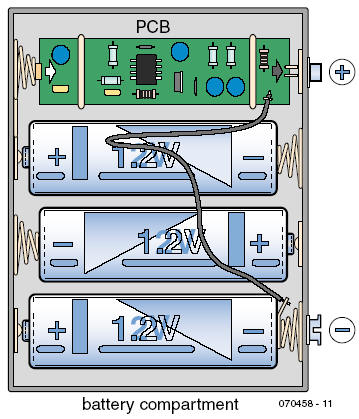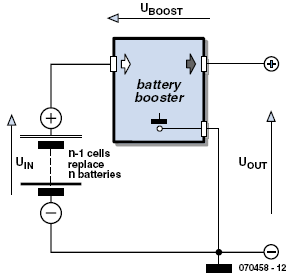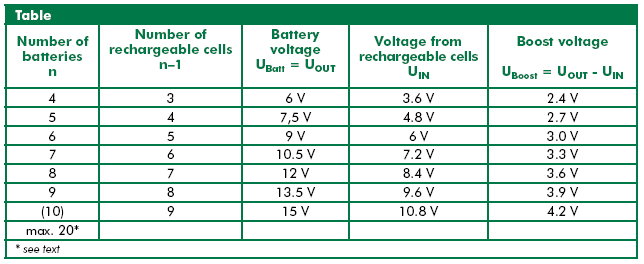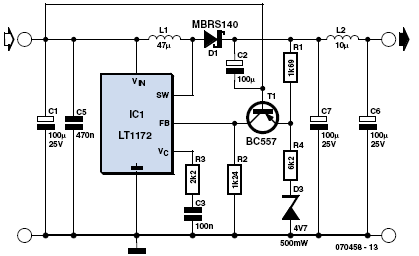Home | Gadget | Fuzzy | Lecturer  | Power  | Transceiver Find more type here... Battery Booster Ralf Schmiedel 1/2008 elektor     The inspiration for this design came from the author’s experience with a mini model helicopter (from Silver lit). This particular model has a hand-held transmitter powered by six AA batteries which acts as a charging station in between flights to recharge the helicopter’s LiPo battery. Even alkaline batteries become discharged relatively quickly because of the energy demands of the helicopter. Replacing the alkaline cells with six rechargeable NiMH batteries brought its own problems; the cell voltage is around 1.4 V after recharging but this quickly levels-out to 1.2 V once you begin drawing energy and this proved to be too low to recharge the helicopter battery. What is needed here is a voltage converter design small enough to fit into the space taken up by an AA battery which pumps up the voltage from the (now five rechargeable cells) up to the level produced by six alkaline batteries. The author was not satisfied with the most simple design solution to the problem; it would be more useful if this booster cell could be used in any battery compartment irrespective of the number of cells. The number of batteries (n) would then be replaced by n–1 rechargeable cells (with one cell position taken up by the booster) giving an output voltage the same as if n primary cells were fitted. The circuit described here can be used in applications requiring four to ten primary cells. With the booster fitted, only three to nine rechargeable cells would be required. The use of (more bulky) electrolytic capacitors with a 35 V rating would allow the booster to be used in applications of up to 20 batteries. In principle almost any switching regulator IC can be used in this way. The power output from this circuit with a LT1172 regulator is around 500 mA but it can be increased to 2 A for example by using the LT1170 instead. Figure 1 shows the circuit built on a small piece of prototyping PCB used in a four-cell battery compartment. The battery booster should always be fitted in the most positive cell position in the compartment (see Figure 2).Figure 1. The booster fits in place of one rechargeable battery and boosts the output voltage up to the equivalent of four alkaline batteries (4 x 1.5 V = 6 V). Figure 2. The Battery Booster operating principle.Figure 3. The circuit diagram and simulation files are available from website at www.elektor.com   The remaining battery positions can then be fitted with NiMH rechargeable cells. The booster circuit produces a voltage UBoost, which is added to the input voltage UIN to give UOUT. The table (below) indicates the necessary boost voltage relative to the number of cells used. The maximum number of cells is limited by the voltage rating of the electrolytic capacitors. The LT1172 itself is rated up to 36 V but to save space 16 V electrolytics are fitted which limits the output to 13.5 V (8 rechargeable cells replacing 9 alkaline cells).   To calculate the value of the boost voltage required UBoost: UBoost = 2.4 V + (0.3 V * (n – 3)) where n = 4 to 10 rechargeable cells.  Or: UBoost = 2.4 V + (UIN – 3.6 V) * 0.3 V / 1.2 V The output voltage is given by: UOUT = UIN + UBoost   The author has used the simulation software Spice and SwitcherCad III (Freeware from LT) with the design and the LTSpice und ASC files from SwitcherCad III are available to download free from the Elektor website so that any interested reader can simulate and modify the design. The Spice model includes inductor L3 which is not shown in the circuit diagram (Figure 3) this simulates the earth wire inductance. A ferrite bead can be used on this lead. The LT1172 operates in this design as a buck-converter. In contrast to the typical configuration where a potential divider network at the output UOUT is be used to produce a feedback voltage to the regulator, here the boost voltage UBoost (see Figure 2) is regulated. Transistor T1 together with R1 forms a current source where output current is proportional to the voltage difference (UBoost–0.7 V)/ R1. As long as the voltage at the emitter of T1 is below the reverse breakdown voltage of the zener diode D3, all of the current will flow through R2 to ground. The resulting voltage drop across R2 is referenced to the 1.24 V reference in the LT1172. With R2 equal to 1.24 kΩ a current of 1 mA flows through R1, T1 and R2 when the circuit is in balance. This current produces a 1.69 V drop across R1 which together with the 0.7 V base-emitter voltage UBE gives a UBoost of 2.4 V, the voltage necessary in a 4-cell application using three rechargeable cells plus the booster (see Table). With this number of cells the circuit operation corresponds to the negative-to-positive buck-boost converter configuration shown in the LT1172 data sheet. For each additional cell the value of UBoost must now be increased by 0.3 V and this is achieved by D3 and R4. When the emitter voltage of T1 is greater than 4.3 V (the sum of UIN and UBE) D3 conducts and a proportion of the current through R1 is diverted through R4 and D3. The voltage across R4 will be the input voltage Uin above 3.6 V, the level produced by three rechargeable cells. The value of R4 can be calculated so that for every additional 1.2 V increase in UIN the UBoost level increases by 0.3 V or 25 % (0.3 V / 1.2 V × 100). UBoost is UR1 + 0.7 V. To increase UBoost by 0.3 V, this voltage must also be dropped across R1. To find the value of R4: n × 0.3 V / 1.69 kΩ = n × 1.2 V / R4 n can be cancelled giving: R4 = 1.2 V / 0.3 V × 1.69 kΩ = 6.8 kΩ So R4 = 6.8 kΩ and   UZ = 4.3 V.   In the simulation UZ was chosen as 4.3 V and R4 as 6.2 kΩ to improve the output voltage level for this application. (A 4.3 V zener is not listed in the SwCad library). As mentioned earlier, different types of buck regulator ICs can be used in a similar configuration. Some types can for example allow the circuit to operate from just a single cell while others supply higher output current. The battery booster can be turned off with a switch in the earth lead.